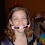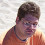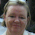## Tuesday, July 31, 2007

### What's Wrong With That?

The following is what seems to be a mathematical proof that ten equals 9.999999.... What's wrong with it?

a = 9.999999...
10a = 99.999999...
10a - a = 90
9a = 90
a = 10

1.The problem comes when you multiply by 10 and subtract. Since 9.999999... is not a rational number (that is, you can't express it in closed form with a fraction, like you could with, say 1.111111... = 10/9), we can only express it to some degree of accuracy (some number of significant digits, that is). Then, when you multiply by 10, the level of precision you chose is moved to the next level. If you do it this way, the problem resolves itself. For example:

a = 9.999
10a = 99.99
10a - a = 89.991
9a = 89.991
a = 9.999

The same principle applies for any number of significant figures you choose.

2.I always thought that this was a legitimate proof, and so the 1 in 89.991 was just a condition of not extending the ...999's.

If this proof is invalid, what does that say about the following:

1/3 + 2/3 = 1
1/3 = .33333...
2/3 = .66666...
.33333... + .66666... = .99999...
Therefore .99999... = 1

?

3.this can also be displayed in the following pattern
1/9= .111111...
2/9= .222222...
3/9= .333333...
...
8/9= .888888...
follwoing this logic,
9/9= .999999... , where the fraction 9/9 also = 1

4.I'm going to side with anonymous and nick on this one. There's (I believe) nothing wrong with the equations. 9.999... is equal to 10.

It was a trick question.

5.I researched it, and it looks like you're right.

9.99999... is an infinite geometric sum:

9*(1/10)^0 + 9*(1/10)^1 + 9*(1/10)^2 = 9 / (1 - 1/10) = 10.

I used the definition of geometric sums, a/(1-r). I still say that level of precision argument I pointed out is sort of the crux of the trick in your proof, but apparently 9.99999.. = 10 in our decimal system for some reason. Good post, Mike.

6.Yeah. I was a bit worried when I saw Abe's post, but other peoples' took me back to reality and reminded me that, yes, this is the same logic as the 0.999... = 1 proof

could the blog author please come forward and let us know whether this was a trick question or whether they just screwed up on this one?

7.Hello DJ Lower/kkairos, I am the blog author and I believe it is a trick question. I've been wrong enough in the past not to be completely certain about that.

8.It definitely is a trick question!
That is one of the extraordinary and magnificient features of real numbers. You can't tell which is the closest smaller number than 10, since 9,999... = 10.

9.sorry, 9.999...=10 :)
I am Finnish and we use "," as the decimal separator

10.Abe's response is the correct. The principal difference is the type of number: rationale or non-rationale. You can't mix orange and lemons :):):)
When we say 1/3=0.333333..., that is an non-rationale aproximation. The correct is 1/3. The difference is minimum (correct) but there is a difference.
So, when you're talk about "aproximation" the equations are correct: "The rationale approximation of the non-rationale 0.999999... is 1"

11.we can prove that 9.99999...... is a rational number and all other numbers in this format (repeated form) are rational numbers
a = 9.999999...
10a = 99.999999...
10a - a = 90
9a = 90
a = 10
by Zafarullah khan

Leave your answer or, if you want to post a question of your own, send me an e-mail. Look in the about section to find my e-mail address. If it's new, I'll post it soon.

Please don't leave spam or 'Awesome blog, come visit mine' messages. I'll delete them soon after.

Enter your Email and join hundreds of others who get their Question of the Day sent right to their mailbox

The Lamplight Manor Puzz 3-D